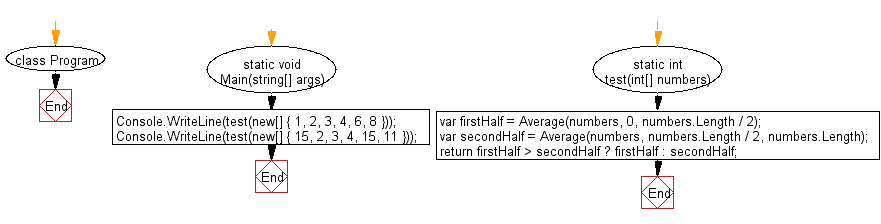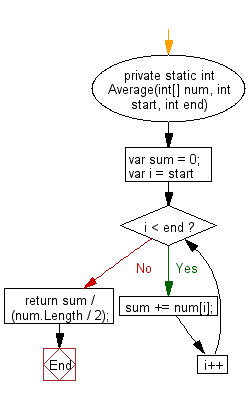﻿ C# - Average value between first, second half of an array# C# Sharp Basic Algorithm Exercises: Find the larger average value between the first and the second half of a given array of integers

## C# Sharp Basic Algorithm: Exercise-135 with Solution

Write a C# Sharp program to find the larger average value between the first and the second half of a given array of integers and minimum length is at least 2. Assume that the second half begins at index (array length)/2.

Sample Solution:

C# Sharp Code:

``````using System;
using System.Collections;
using System.Collections.Generic;
using System.Linq;
using System.Text;
namespace exercises
{
class Program
{
static void Main(string[] args)
{
Console.WriteLine(test(new[] { 1, 2, 3, 4, 6, 8 }));
Console.WriteLine(test(new[] { 15, 2, 3, 4, 15, 11 }));
}
static int test(int[] numbers)
{
var firstHalf = Average(numbers, 0, numbers.Length / 2);
var secondHalf = Average(numbers, numbers.Length / 2, numbers.Length);
return firstHalf > secondHalf ? firstHalf : secondHalf;
}

private static int Average(int[] num, int start, int end)
{
var sum = 0;
for (var i = start; i < end; i++)
sum += num[i];
return sum / (num.Length / 2);
}

}
}
```
```

Sample Output:

```6
10
```

Flowchart: 1Flowchart: 2C# Sharp Code Editor:

Improve this sample solution and post your code through Disqus

What is the difficulty level of this exercise?

Test your Programming skills with w3resource's quiz.

﻿